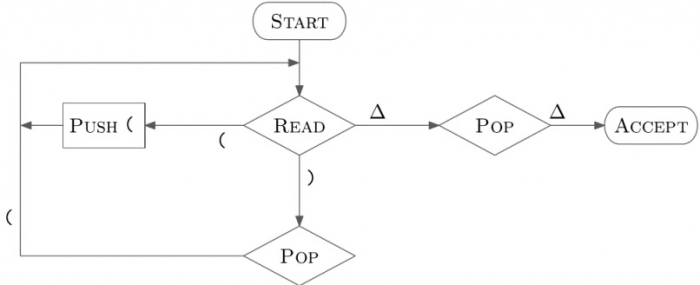# Explain the balancing parenthesis of PDA

Data Structure AlgorithmsComputer ScienceComputers

Pushdown Automata (PDA) are the finite automata (FAs), but with the ability to push and pop symbols to/from a stack.

PDA accepts strings if there is a legal path from start state to acceptance state for input. Otherwise, the string is rejected.

A PDA can be represented by a 7-tuple

(Q, ∑, ℾ, q0, ha, ∆,δ)

Where

The PDA is to finite subsets of Q ☓ (ℾ ∪ {∆})*.

## Parentheses are balanced if

• While reading string, number of opening parentheses >= number of closing parentheses.
• When string is read, number of opening parentheses = number of closing parentheses.

## Examples

• (())() − Balanced
• ((()() − Not balanced
• )()(() − Not balanced

The context free grammar (CFG) is as follows −

S -> (S) | SS | ε

## PDA for balancing brackets

Each (is pushed, each) causes an (to be popped). This is shown below